# 带你读 MySQL 源码：where 条件怎么过滤记录？

0收藏

• 1. 准备工作
• 2. 整体介绍
• 3. 源码分析

○ 3.1 ExecuteIteratorQuery()

○ 3.3 compare_int_signed()

○ 3.4 Arg_comparator::compare()

○ 3.5 Item_func_gt::val_int()

○ 3.6 Item_cond_and::val_int()

○ 3.7 Item_func_eq::val_int()

○ 3.8 Item_cond_or::val_int()

• 4. 总结

### 1. 准备工作

``````CREATE TABLE `t1` (
`id` int unsigned NOT NULL AUTO_INCREMENT,
`str1` varchar(255) DEFAULT '',
`i1` int DEFAULT '0',
`i2` int DEFAULT '0',
PRIMARY KEY (`id`) USING BTREE
) ENGINE=InnoDB DEFAULT CHARSET=utf8mb3;``````

``````INSERT INTO t1(str1, i1, i2) VALUES
('s1', NULL, NULL),
('s2', 20, NULL),
('s3', 30, 31),
('s4', 40, 41),
('s5', 50, 51),
('s6', 60, 61),
('s7', 70, 71),
('s8', 80, 81);``````

``````select * from t1
where i2 > 20 and (i1 = 50 or i1 = 80)``````

### 2. 整体介绍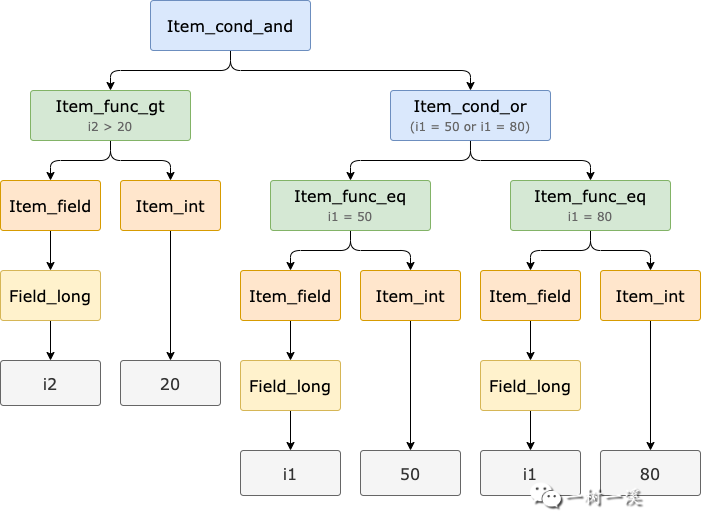• Item_cond_and 代表 where 条件中的​`​and​`​，连接 Item_func_gt 和 Item_cond_or。
• Item_func_gt 代表​`​i2 > 20​`​，其中 Item_field 包含 Field_long，代表 i2 字段，Item_int 代表整数 20。
• Item_cond_or 代表 where 条件中的​`​or​`​，连接两个 Item_func_eq。
• 第 1 个 Item_func_eq 代表​`​i1 = 50​`​，其中 Item_field 包含 Field_long，代表 i1 字段，Item_int 代表整数 50。
• 第 2 个 Item_func_eq 代表​`​i1 = 80​`​，其中 Item_field 包含 Field_long，代表 i1 字段，Item_int 代表整数 80。

``````| > mysql_execute_command(THD*, bool) sql/sql_parse.cc:4688
| + > Sql_cmd_dml::execute(THD*) sql/sql_select.cc:578
| + - > Sql_cmd_dml::execute_inner(THD*) sql/sql_select.cc:778
| + - x > Query_expression::execute(THD*) sql/sql_union.cc:1823
| + - x = > Query_expression::ExecuteIteratorQuery(THD*) sql/sql_union.cc:1770
| + - x = | > FilterIterator::Read() sql/iterators/composite_iterators.cc:79
| + - x = | + > Item_cond_and::val_int() sql/item_cmpfunc.cc:5973
| + - x = | + - > // 第 1 个 Item::val_bool()
| + - x = | + - > // 代表 i2 > 20
| + - x = | + - > Item::val_bool() sql/item.cc:218
| + - x = | + - x > Item_func_gt::val_int() sql/item_cmpfunc.cc:2686
| + - x = | + - x = > Arg_comparator::compare() sql/item_cmpfunc.h:210
| + - x = | + - x = | > Arg_comparator::compare_int_signed() sql/item_cmpfunc.cc:1826
| + - x = | + - x = | + > Item_field::val_int() sql/item.cc:3013
| + - x = | + - x = | + - > Field_long::val_int() const sql/field.cc:3763 // i2
| + - x = | + - x = | + > Item_int::val_int() sql/item.h:4934 // 20
| + - x = | + - > // 第 2 个 Item::val_bool()
| + - x = | + - > // 代表 i1 = 50 or i1 = 80
| + - x = | + - > Item::val_bool() sql/item.cc:218
| + - x = | + - x > Item_cond_or::val_int() sql/item_cmpfunc.cc:6017
| + - x = | + - x = > // 第 3 个 Item::val_bool()
| + - x = | + - x = > // 代表 i1 = 50
| + - x = | + - x = > Item::val_bool() sql/item.cc:218
| + - x = | + - x = | > Item_func_eq::val_int() sql/item_cmpfunc.cc:2493
| + - x = | + - x = | + > Arg_comparator::compare() sql/item_cmpfunc.h:210
| + - x = | + - x = | + - > Arg_comparator::compare_int_signed() sql/item_cmpfunc.cc:1826
| + - x = | + - x = | + - x > Item_field::val_int() sql/item.cc:3013
| + - x = | + - x = | + - x = > Field_long::val_int() const sql/field.cc:3763 // i1
| + - x = | + - x = | + - x > Item_int::val_int() sql/item.h:4934 // 50
| + - x = | + - x = > // 第 4 个 Item::val_bool()
| + - x = | + - x = > // 代表 i1 = 80
| + - x = | + - x = > Item::val_bool() sql/item.cc:218
| + - x = | + - x = | > Item_func_eq::val_int() sql/item_cmpfunc.cc:2493
| + - x = | + - x = | + > Arg_comparator::compare() sql/item_cmpfunc.h:210
| + - x = | + - x = | + - > Arg_comparator::compare_int_signed() sql/item_cmpfunc.cc:1826
| + - x = | + - x = | + - x > Item_field::val_int() sql/item.cc:3013
| + - x = | + - x = | + - x = > Field_long::val_int() const sql/field.cc:3763 // i1
| + - x = | + - x = | + - x > Item_int::val_int() sql/item.h:4934 // 80``````

• 第 1 个 Item::val_bool() 代表​`​i2 > 20​`​​，经过多级调用 Arg_comparator::compare_int_signed() 判断记录的 i2 字段值是否​`​大于​`​ 20。
• 第 2 个 Item::val_bool() 代表​`​i1 = 50 or i1 = 80​`​。

• 第 3 个 Item::val_bool() 代表​`​i1 = 50​`​​，经过多级调用 Arg_comparator::compare_int_signed() 判断记录的 i1 字段值是否​`​等于​`​ 50。
• 第 4 个 Item::val_bool() 代表​`​i1 = 80​`​​，经过多级调用 Arg_comparator::compare_int_signed() 方法判断记录的 i1 字段值是否​`​等于​`​ 80。

### 3. 源码分析

#### 3.1 ExecuteIteratorQuery()

``````// sql/sql_union.cc
bool Query_expression::ExecuteIteratorQuery(THD *thd){
...
{
...
for (;;) {
// 从存储引擎读取一条记录
DBUG_EXECUTE_IF("bug13822652_1", thd->killed = THD::KILL_QUERY;);

// 读取出错，直接返回
if (error > 0 || thd->is_error())  // Fatal error
return true;
// error < 0
// 表示已经读完了所有符合条件的记录
// 查询结束
else if (error < 0)
break;
// SQL 被客户端干掉了
else if (thd->killed)  // Aborted by user
{
thd->send_kill_message();
return true;
}
...
// 发送数据给客户端
if (query_result->send_data(thd, *fields)) {
return true;
}
...
}
}
...
}``````

m_root_iterator->Read() 从存储引擎读取一条记录，对于示例 SQL 来说，m_root_iterator 是 FilterIterator 迭代器对象，实际执行的方法是 ​`​FilterIterator::Read()​`​。

``````int FilterIterator::Read(){
for (;;) {
if (err != 0) return err;

bool matched = m_condition->val_int();

if (thd()->killed) {
thd()->send_kill_message();
return 1;
}

/* check for errors evaluating the condition */
if (thd()->is_error()) return 1;

if (!matched) {
m_source->UnlockRow();
continue;
}

// Successful row.
return 0;
}
}``````

m_source->Read() 方法从存储引擎读取一条记录，因为示例 SQL 中 t1 表的访问方式为​`​全表扫描​`​，所以 m_source 是 TableScanIterator 迭代器对象。

``````explain select * from t1
where i2 > 20 and (i1 = 50 or i1 = 80)\G

***************************[ 1. row ]***************************
id            | 1
select_type   | SIMPLE
table         | t1
partitions    | <null>
type          | ALL
possible_keys | <null>
key           | <null>
key_len       | <null>
ref           | <null>
rows          | 8
filtered      | 12.5
Extra         | Using where``````

m_condition 代表 SQL 的 where 条件，对于示例 SQL 来说，它是 Item_cond_and 对象。

m_condition->val_int() 实际执行的方法是 ​`​Item_cond_and::val_int()​`​，这就是判断记录是否匹配示例 SQL where 条件的入口。

#### 3.3 compare_int_signed()

``````// sql/item_cmpfunc.cc
int Arg_comparator::compare_int_signed(){
// 获取 where 条件操作符左边的值
// 例如：i2 > 20
// 获取当前读取记录的 i2 字段值
longlong val1 = (*left)->val_int();
if (current_thd->is_error()) return 0;
// where 条件操作符左边的值不为 NULL
// 才进入 if 分支
if (!(*left)->null_value) {
// 获取 where 条件操作符右边的值
// 例如：i2 > 20
// val2 的值就等于 20
longlong val2 = (*right)->val_int();
if (current_thd->is_error()) return 0;
// where 条件操作符右边的值不为 NULL
// 才进入 if 分支
if (!(*right)->null_value) {
// 到这里，where 条件操作符左右两边的值都不为 NULL
// 把 where 条件的 null_value 设置为 false
if (set_null) owner->null_value = false;
// 接下来 3 行代码
// 比较 where 条件操作符左右两边的值的大小
if (val1 < val2) return -1;
if (val1 == val2) return 0;
return 1;
}
}
// 如果执行到下面这行代码
// 说明 where 条件操作符左右两边的值
// 至少有一个是 NULL
// 把 where 条件的 null_value 设置为 true
if (set_null) owner->null_value = true;
return -1;
}``````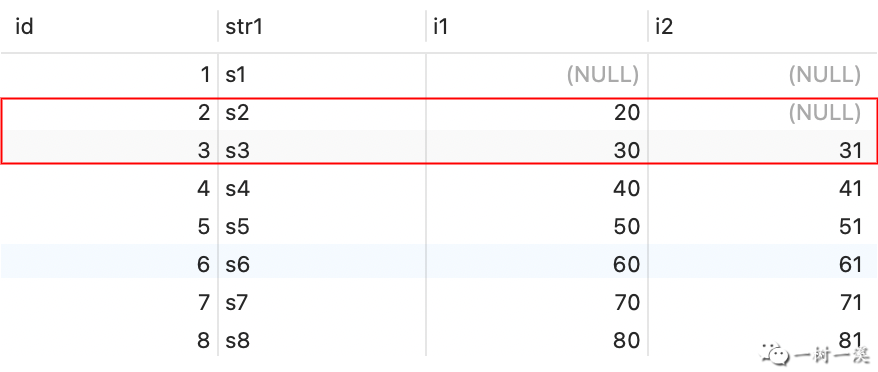i2 字段值为 NULL，​`​if (!(*left)->null_value)​`​​ 条件不成立，执行流程直接来到 ​`​if (set_null) owner->null_value = true​`​，把 where 条件的 null_value 设置为 true，表示对于当前读取的记录，where 条件包含 NULL 值。

i2 字段值为 31（即 val1 = 31），​`​if (!(*left)->null_value)​`​ 条件成立，执行流程进入该 if 分支。

• `​if (set_null) owner->null_value = false​`​，把 where 条件的 null_value 设置为 false，表示对于当前读取的记录，where 条件不包含 NULL 值。
• `​if (val1 < val2)​`​，val1 = 31 大于 val2 = 20，if 条件不成立。
• `​if (val1 == val2)​`​，val1 = 31 大于 val2 20，if 条件不成立。
• `​return 1​`​，因为 val1 = 31 大于 val2 = 20，返回 1，表示当前读取的记录匹配 where 条件 i2 > 20。

#### 3.4 Arg_comparator::compare()

``````// sql/item_cmpfunc.h
inline int compare(){ return (this->*func)(); }``````

Arg_comparator::compare() 只有一行代码，就是调用 ​`​*func​`​ 方法，比较两个值的大小。

func 属性保存了用于比较两个值大小的方法的地址，在 ​`​Arg_comparator::set_cmp_func(...)​`​ 中赋值。

#### 3.5 Item_func_gt::val_int()

``````// sql/item_cmpfunc.cc
longlong Item_func_gt::val_int(){
assert(fixed == 1);
int value = cmp.compare();
return value > 0 ? 1 : 0;
}``````

• `​-1​`​​：表示 where 条件操作符左边的值​`​小于​`​右边的值。
• `​0​`​​：表示 where 条件操作符左边的值​`​等于​`​右边的值。
• `​1​`​​：表示 where 条件操作符左边的值​`​大于​`​右边的值。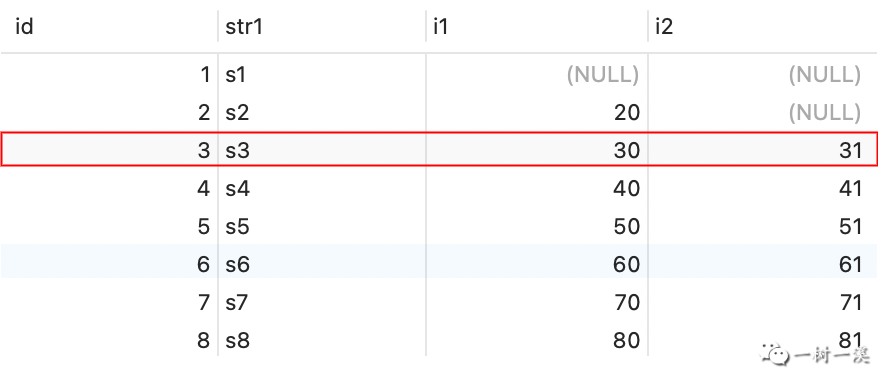i2 字段值为 31，对 where 条件 i2 > 20 调用 cmp.compare()，得到的返回值为 1（即 value = 1）。

`​value > 0 ? 1 : 0​`​ 表达式的值为 1，这就是 Item_func_ge::val_int() 的返回值，表示 id = 3 的记录匹配 where 条件 i2 > 20。

#### 3.6 Item_cond_and::val_int()

``````// sql/item_cmpfunc.cc
longlong Item_cond_and::val_int(){
assert(fixed == 1);
// and 连接的 N 个 where 条件都保存到 list 中
// 根据 list 构造迭代器
List_iterator_fast<Item> li(list);
Item *item;
null_value = false;
// 迭代 where 条件
while ((item = li++)) {
if (!item->val_bool()) {
if (ignore_unknown() || !(null_value = item->null_value))
return 0;  // return false
}
if (current_thd->is_error()) return error_int();
}
return null_value ? 0 : 1;
}``````

Item_cond_and::val_int() 的逻辑：

• 判断当前读取的记录是否匹配 Item_cond_and 对象所代表的 and 连接的 N 个 where 条件（N >= 2）。
• 如果对​`​每个​`​​条件调用 item->val_bool() 的返回值​`​都是​`​ true，说明记录匹配 and 连接的 N 个 where 条件。
• 如果对​`​某一个或多个​`​条件调用 item->val_bool() 的返回值是 false，就说明记录不匹配 and 连接的 N 个 where 条件。

null_value 的初始值被设置为 false，表示 and 连接的 N 个 where 条件中，还没出现哪个 where 条件包含 NULL 值的情况（毕竟还啥都没干）。

null_value 比较重要，它有可能最终决定 Item_cond_and::val_int() 的返回值（后面会介绍）。

while 循环迭代 and 连接的 N 个 where 条件。

if (current_thd->is_error())，这行代码表示执行过程中出现了错误，我们先忽略它。

``````class Item_cond : public Item_bool_func {
...
/// Treat UNKNOWN result like FALSE
/// because callers see no difference
bool ignore_unknown() const { return abort_on_null; }
...
}``````

• ignore_unknown()
• !(null_value = item->null_value))

• null_value = item->null_value
• !null_value

item->null_value = false，表示对于当前读取的记录，where 条件​`​不包含​`​ NULL 值。

item->null_value = true，表示对于当前读取的记录，where 条件​`​包含​`​ NULL 值。

while 循环迭代完 and 连接的 N 个 where 条件之前，如果 Item_cond_and::val_int() 方法的执行流程都没有被 while 代码块中包含的 ​`​return 0​`​​ 提前结束，执行流程就会来到 ​`​return null_value ? 0 : 1​`​。

> 场景 1

while 循环迭代 and 连接的 N 个 where 条件的过程中，对每个条件调用 item->val_bool() 的返回值都是 true。

> 场景 2

while 循环迭代 and 连接的 N 个 where 条件的过程中，某个条件​`​同时满足​`​以下 4 个要求：

• 调用 item->val_bool() 的返回值是 false，说明当前读取的记录不匹配该条件。
• ignore_unknown() 的返回值也是 false，表示包含 NULL 值的 where 条件的比较结果（​`​UNKNOWN​`​）不按 false 处理，而是要等到 while 循环结束之后，根据 null_value 属性的值（true 或 false）算总帐。

• !(null_value = item->null_value)) 表达式的值为 false，说明该条件包含 NULL 值，那么它就是 ignore_unknown() = false 时需要等到 while 循环结束之后，根据 null_value 属性的值算总帐的条件。
• 该条件之后的其它 where 条件，不会导致 while 循环被提前中止（这样执行流程才能来到​`​return null_value ? 0 : 1​`​）。

#### 3.7 Item_func_eq::val_int()

``````// sql/item_cmpfunc.cc
longlong Item_func_eq::val_int(){
assert(fixed == 1);
int value = cmp.compare();
return value == 0 ? 1 : 0;
}``````

• `​-1​`​​：表示 where 条件操作符左边的值​`​小于​`​右边的值。
• `​0​`​​：表示 where 条件操作符左边的值​`​等于​`​右边的值。
• `​1​`​​：表示 where 条件操作符左边的值​`​大于​`​右边的值。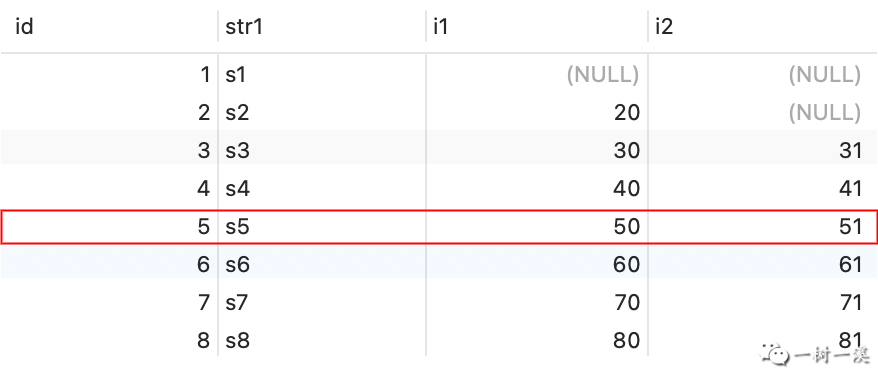i1 字段值为 50，对 where 条件 i1 = 50 调用 cmp.compare()，得到的返回值为 0（即 value = 0）。

`​value == 0 ? 1 : 0​`​ 表达式的值为 1，这就是 Item_func_eq::val_int() 的返回值，表示 id = 5 的记录匹配 where 条件 i1 = 50。

#### 3.8 Item_cond_or::val_int()

``````// sql/item_cmpfunc.cc
longlong Item_cond_or::val_int(){
assert(fixed == 1);
List_iterator_fast<Item> li(list);
Item *item;
null_value = false;
while ((item = li++)) {
if (item->val_bool()) {
null_value = false;
return 1;
}
if (item->null_value) null_value = true;
...
}
return 0;
}``````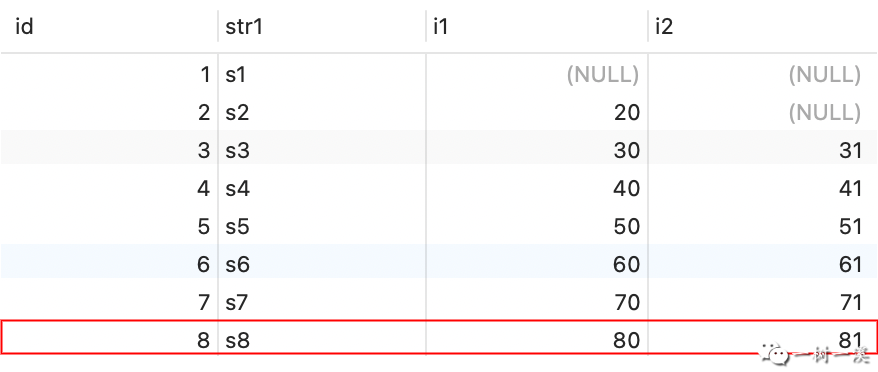Item_cond_or 对象的 list 属性包含 2 个条件：i1 = 50、i1 = 80，List_iterator_fastli(list) 根据 list 构造一个迭代器。

if (item->null_value) 条件不成立，​`​不执行​`​ null_value = true。

`​return 1​`​，这就是 Item_cond_or::val_int() 的返回值，表示 id = 8 的记录匹配 where 条件 i1 = 50 or i1 = 80。

### 4. 总结

• 从存储引擎读取一条记录之后，对 and 连接的 N 个 where 条件（N >= 2）调用 item->val_bool() 的返回值​`​必须全部等于​`​​ true，记录才匹配 and 连接的 N 个 where 条件。
Item_cond_and::val_int() 的代码不多，但是这个方法中调用了​​`​ignore_known()​`​ 用于控制怎么处理 where 条件包含 NULL 值的场景，代码细节并不太好理解，所以花了比较长的篇幅介绍 Item_cond_and::val_int() 方法的逻辑，需要多花点时间去理解其中的逻辑。
• 从存储引擎读取一条记录之后，对 or 连接的 N 个 where 条件（N >= 2）调用 item->val_bool()，只要​`​其中一个​`​返回值等于 true，记录就匹配 or 连接的 N 个 where 条件。相关问题
社区精华内容